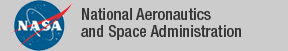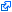### Top File of PVS digraphs Library

```
%  min_walk_reduced      -- theorem that minimum walk is reduced
%  min_walks             -- minimum walk satisfying a propertyx
%  path_ops              -- deleting vertex and edge operations
%  paths                 -- fundamental definition and properties about paths
%  reduce_walks          -- operation to reduce a walk
%  sep_sets              -- definition of separating sets
%  di_subgraphs           -- generation of di_subgraphs from vertex sets
%  di_subgraphs_from_walk -- generation of di_subgraphs from walks
%  subtrees              -- subtrees of a digraph
%  trees                 -- fundamental definition of trees
%  walk_inductions       -- induction on length of a walk
%  walks                 -- fundamental definition and properties of walks
%
%------------------------------------------------------------------------------
top: THEORY

BEGIN

IMPORTING digraphs,
digraph_deg,
walks,
paths,
path_ops,
dags,
circuits,
cycles,
di_subgraphs,
di_subgraphs_from_walk,
digraph_ops,
max_di_subgraphs,
max_subtrees,
trees,
subtrees,
walk_inductions,
min_walk_reduced,
reduce_walks,
abstract_min,
abstract_max,
pairs,
digraph_conn_defs,
digraph_inductions,
ind_paths,
min_walks,
sep_sets,
weighted_digraphs,
wgt_digraphs_props,
Eulerian

%  digraph_deg_sum       -- theorem relating vertex degree and number of edges
%  tree_circ             -- theorem that tree has no circuits
%  digraph_connected     -- all connected defs are equivalent
%  ramsey_new            -- Ramsey's theorem
%  menger                -- menger's theorem
%  tree_paths            -- theorem that tree has only one path between vertices
%  circuit_deg           -- degree of circuits
%  cycle_deg             -- theorem about degree and existence of cycle
%  digraph_complected    -- unusual definition of connected digraph
%  digraph_conn_piece    -- structural connected ==> piece connected
%  digraph_path_conn     -- path connected ==> structural connected
%  digraph_piece_path    -- piece connected ==> path connected
%  h_menger              -- hard menger
%  meng_scaff            -- scaffolding for hard menger proof
%  meng_scaff_defs       -- scaffolding for hard menger proof
%  meng_scaff_prelude    -- scaffolding for hard menger proof
%  sep_set_lems          -- properties of separating sets

%            digraph_deg_sum,
%            tree_circ,
%            digraph_connected,
%            ramsey_new,
%            menger,
%            tree_paths,         % Proof incorrect
%            circuit_deg,
%	     cycle_deg,          % not attempted yet
%	     digraph_complected  ,
%	     digraph_conn_piece  ,
%	     digraph_connected   ,
%	     digraph_path_conn   ,
%	     digraph_piece_path  ,
%	     h_menger          ,
%	     meng_scaff        ,
%	     meng_scaff_defs   ,
%	     meng_scaff_prelude,
%	     menger            ,
%	     sep_set_lems      ,

END top

```
The tagidentifies links that are outside the NASA domain